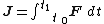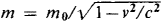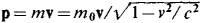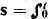# impulse

(redirected from Impulse (mechanics))
Also found in: Dictionary, Thesaurus, Medical.

## impulse,

in mechanics: see momentummomentum
, in mechanics, the quantity of motion of a body, specifically the product of the mass of the body and its velocity. Momentum is a vector quantity; i.e., it has both a magnitude and a direction, the direction being the same as that of the velocity vector.
.

## Impulse (mechanics)

The integral of a force over an interval of time. For a force F , the impulse J over the interval from t0 to t1 can be written as Eq. (1).

(1)The impulse thus represents the product of the time interval and the average force acting during the interval. Impulse is a vector quantity with the units of momentum.

The momentum-impulse relation states that the change in momentum of a mass m over a given time interval equals the impulse of the resultant force acting during that interval. The momentum change can be expressed in terms of the velocities v 1 and v 0 at times t1 and t0, respectively, giving Eq. (2). (2)See Impact, Momentum

McGraw-Hill Concise Encyclopedia of Physics. © 2002 by The McGraw-Hill Companies, Inc.
The following article is from The Great Soviet Encyclopedia (1979). It might be outdated or ideologically biased.

## Impulse

(in Russian, impul’s). (1) Mechanical impulse is a measure of mechanical motion; it is a vector quantity that for a mass particle is equal to the product of the mass m of the particle and its velocity v and has the same direction as the velocity vector: p = mv; the same as momentum. [In Russian the terms impul’s and kolichestvo dvizheniia are used interchangeably to mean “momentum”; the term impul’s sily denotes “impulse” as understood in American usage. See alsoIMPULSEandMOMENTUM.] For a particle moving with a speed close to the speed of light in a vacuum c, the dependence of its mass on velocity must be taken into account:, where m0 is the mass of a particle at rest (the so-called rest mass). In this case the momentum of a free particle is, and the relationship between the total energy ε of a particle and its impulse is given by the relation ε2 = p2c2 + m02c4. For a particle with zero rest mass (such as a photon or neutrino), p = ε/c; such particles always move at the speed of light c.

All forms of matter, including electromagnetic and gravitational fields, have momentum. The momentum density vector (the momentum per unit volume occupied by the field), which is expressed in terms of field intensity, potentials, and so on, is introduced for fields.

(2) A wave impulse is a single perturbation that is propagating in space or in a medium. An example of such an impulse is a sound pulse (such as the sound of a pistol shot), which is an abrupt and rapidly vanishing rise in pressure that produces a wave front of a short-lived increase in pressure that propagates from the place of the disturbance. Such as isolated pulse consists of all the frequency components of a continuous spectrum, from the lowest to those whose period is close to the duration of the pulse. Such a sound pulse is used to determine the frequency characteristics of receivers, as well as in architectural acoustics to detect echoes and to determine the reverberation time in buildings.

Another example of a wave pulse is an electromagnetic perturbation that propagates from the place of a rapid change in an electric or magnetic field induced, for example, by a strong spark, lightning, or some other pulsed electrical process. The spectrum of such an electromagnetic impulse is also continuous and contains all frequencies from the lowest to the extremely high.

A light pulse is a brief emission of light (0.01 sec or less) by an optical radiation source. The spectral composition of a light pulse is determined by the type of source, which may be a pulsed electrical discharge in gases or the flash that accompanies the explosion of a fine conductor when a strong electric current is passed through it. The short duration of such pulses makes possible the production of high instantaneous values of the light radiation power, reaching 106 kilowatts (kW) in some cases. Light pulses are used to study processes that proceed rapidly (for example, in high-speed still and motion-picture photography and in the photography of the tracks of elementary particles in tracking instruments), for the optical pumping of lasers, in automatic devices having photoelectric control and information channels, and in light signaling equipment.

Pulses are usually used in physics and technology in the form of a short train or group of waves. Such a pulse may be isolated or may be repeated after time intervals longer than or comparable to its duration. A sound signal (pulse) of specific frequency, whose duration is a few periods (10–100), is often used in acoustics. Sonic and ultrasonic pulses are used extensively in hydroacoustical research, especially to measure depth, in hydrolocation, and in ultrasonic flaw detection.

Light pulses, which are short wave trains, may be emitted by lasers operating in the pulsed mode. The impulse may last 10−10 or 10−12 sec, and the power may reach 1 billion kW.

A single, brief surge of electrical current or voltage is called a current or voltage pulse.

## Impulse

a measure of the action of a force over some time interval; it is equal to the product of the average value of the force Fav and the time t1 of its action: S = Favt1. Impulse is a vector quantity and has the same direction as Fav, The exact value of impulse over a time interval t1 is determined by an integral:F dt. When a mass point moves under the action of a force F, its momentum receives over time t1 an increment equal to the impulse:

S = mv0mv1 S = mv0mv1

where mv0 and mv1 are the momentums of the particle at the beginning and end of the time interval t1, respectively.

The concept of impulse is used widely in mechanics, especially in impact theory, where a quantity equal to the impulse of the impact force Fimp over the time of impact τ is called the impact impulse.

## impulse

[′im‚pəls]
(mechanics)
The integral of a force over an interval of time.
(metallurgy)
A single pulse or several pulses in welding current used in resistance welding.
(physics)
A pulse which lasts for so short a time that its duration can be thought of as infinitesimal.
(psychology)
A sudden psychogenic urge to act.
McGraw-Hill Dictionary of Scientific & Technical Terms, 6E, Copyright © 2003 by The McGraw-Hill Companies, Inc.

## impulse

1. Physics
a. the product of the average magnitude of a force acting on a body and the time for which it acts
b. the change in the momentum of a body as a result of a force acting upon it for a short period of time
2. Physiol See nerve impulse
3. Electronics a less common word for pulse
Collins Discovery Encyclopedia, 1st edition © HarperCollins Publishers 2005
Site: Follow: Share:
Open / Close